# (extended) Freeth's nephroid

## strophoid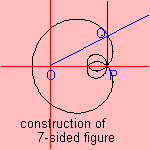The curve, a botanic curve, is an extension of Freeth's nephroid (b = 2). This specimen is the strophoid constructed for a circle, named after the English mathematician T.J. Freeth (1819-1904). The Freeth's nephroid (b=2) can be used to construct a regular 7-sided figure, as follows: Let P be the node where the curve crosses itself three times. Line l parallel the y-axis through P cuts the nephroid in Q. Then the angle POQ is 3π/7.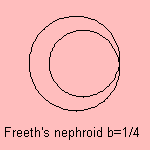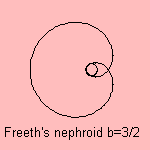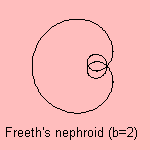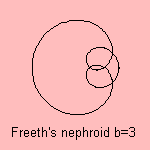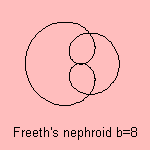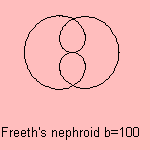For large b the curve approximates the rhodonea (c=1/2). Don't mistake this curve with another nephroid, the (ordinary) nephroid 1). notes 1) Nephros (Greek) = kidney